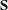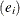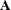# The ACECLUS Procedure

### Displayed Output

Unless the SHORT option is specified, the ACECLUS procedure displays the following items:

• Means and Standard Deviations of the input variables

• thematrix, labeled COV: Total Sample Covariances

• the name or value of the matrix used for the Initial Within-Cluster Covariance Estimate

• the Threshold value if the PROPORTION= option is specified

For each iteration, PROC ACECLUS displays the following items:

• the Iteration number

• RMS Distance, the root mean square distance between all pairs of observations

• the Distance Cutofffor including pairs of observations in the estimate of the within-cluster covariances, which equals the RMS distance times the threshold

• the number of Pairs Within Cutoff

• the Convergence Measureas specified by the METRIC= option

If the SHORT option is not specified, PROC ACECLUS also displays thematrix, labeled ACE: Approximate Covariance Estimate Within Clusters.

The ACECLUS procedure displays a table of eigenvalues from the canonical analysis containing the following items:

• Eigenvalues of Inv(ACE)*(COV–ACE)

• the Difference between successive eigenvalues

• the Proportion of variance explained by each eigenvalue

• the Cumulative proportion of variance explained

If the SHORT option is not specified, PROC ACECLUS displays the following items:

• the Eigenvectors or raw canonical coefficients

• the standardized eigenvectors or standard canonical coefficients